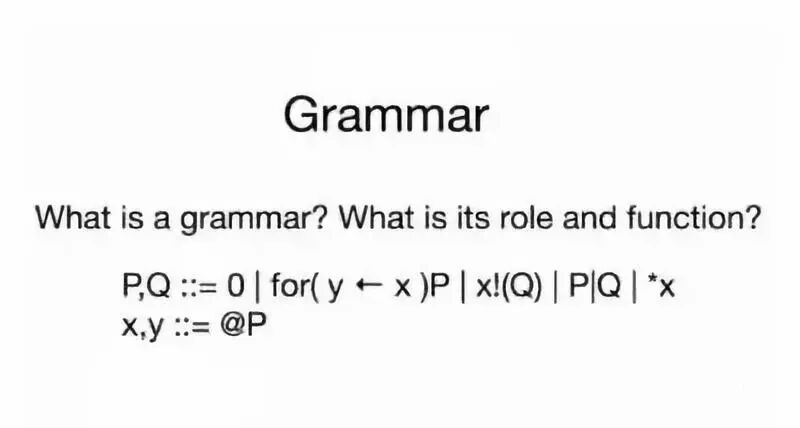# 可计算性理论模型初识（一）：巴斯克范式（BNF）与λ演算

• A+Greg Meredith近期在Youtube上教授一门课程Introduction to the Designof Computational Calculi (DoCC) ，每周更新。主要为了让RChain爱好者们理解可计算理论及其包含的演算模型，详解rho演算是如何应用在RChain项目中并教授大家如何基于该演算模型设计RChain相关应用。

λ演算

λ演算是一种通用的计算模型，由数学家AlonzoChurch在20世纪30年代提出。 用BNF描述λ演算的语法表示如下：

M,N ::= λx.M |(M N)  （1.1）

M[X]::= λX.M[X] | (M[XM[X])

• x（变量;从集合X中选择一个变量，诚然这与M和N没什么关系，变量本身也是个λ项）
• λx.M（抽象;将变量x绑定在M中，即在M中声明x变量，抽象也是λ项）
• （M N）（应用;将 λ项M“应用”到λ项N，应用同样也是λ项）

1．λx.λy.x是符合规范的λ项（这是一个重要的例子，因为它是λ演算中布尔函数True的编码）

2. （λx.xy）是符合规范的λ项（这也是一个重要的例子，因为λx.x充当λ演算中的恒等函数）

3.  xλx。是不正确的语法⇒不是一个可接受的λ项

λ演算可以映射为函数的概念：抽象（λx.M）从一个函数中选择变量，应用（M N）用函数来评估变量。这个思想指导了这些结构间的相互作用，称之为操作语义。在λ演算中主要有两种关系，α-变换和β-规约：

• α-变换

λx.M{x}→ λy.M{y}

• β-规约

（λx.MN）→M {N / x}

β规约主要表达函数的应用。（λx.MN）中，前半部分是λ抽象λx.M，后面的N用于替代λx.M中的x，也即λx.M应用于N。这即是一个简单的函数评估：在函数M中声明变量x并用其评估N，即用N代替x。这也可以被认为是功能组合，如果我们将函数f(x)应用于g(y)，我们可以得到函数f(g(y))。这与替换x=g(y)效果完全相同。

1，λx.（x z）→λy.（y z）

2，（λx.（x x）y）→（y y）

3，（λx.（λy.（x y）u）v）→（v u）

• x：变量声明，int x
• λx.M：函数声明, def M(x)
• （M N）：M（x）和N(y)都是函数，表示M(N(y)), 要求N(y)的结果与变量x声明的类型一致或取值范围一致，此例子中为int型。

α-变换: 函数的内容一致时，修改函数变量名函数的功能是没区别的，f（x）与f（y）等价，α-变换避免了不同函数中变量名称冲突的问题

β-规约：两个函数f(x)和g(y)，当x= g(y)时候，可以表示为f(g(y))

*补充，η-变换：如果两个函数在变量相同时总有f(x)===g(x)，则f=g

 Computational Calculi Primer Part 1:Lambda calculus & BNF (https://blog.rchain.coop/computational-calculi-primer/)

 Wikipedia-Lambda calculus (https://en.wikipedia.org/wiki/Lambda_calculus)